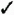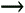# Section 53. Enter Calc Functions

### 53. Enter Calc FunctionsBEFORE YOU BEGINSEE ALSO

54 Use the Function Wizard

Computing totals is so common, Calc makes the Sum() function even easier to use by placing the Sum button on the Formula bar. Just click a blank cell below or to the right of a range of values, click the Sum button, and Calc computes the sum and writes the proper Sum() function for you. If Calc guesses incorrectly at which cells you want to include in the function, you can edit the cell addresses in the Formula bar.

53. Enter Calc FunctionsYou'll have to type the remaining functions yourself when you want to use them, but doing so is far from a chore. 52 About Calc Functions lists some of the most common functions you'll use. For the rest, use Calc's Help feature to find the function you want to use and then type that function and its arguments to get the result you want.

 1 Select the Cell to Hold the Function Click to select the cell you want to contain the results of the function. 2 Request the Function Type an equals sign (=) followed by the name of the function. For the Sum() function, you can bypass this step and simply click the Sum button on the Formula bar. 3 Enter the Arguments to Include Type an open parenthesis ( ( ) and then type or select the values you want to include in the function, followed by a closing parenthesis ( ) ). A border appears around the cell or range to be included in the equation. For the Sum() function, your task is simplified once again because Calc proposes a range as soon as you click the Sum button. If it guesses wrong, drag to adjust the selection. If the range is correct, press Enter to accept the sum; otherwise , type a different range inside the Sum() function's argument list and then press Enter . The sum then appears in the cell.TIP The Status bar at the bottom of the work area provides a quick way to see the result of common functions on-the-fly . Simply select a range, and the status bar automatically displays the range's sum until you select a different cell or range. You can right-click in the Status bar to automatically display other common functions such as Average or Count , or to display no results at all if you don't want this quick reference available as you work.NOTE As with formulas, you can select a cell containing a function and press F2 to change the function, its arguments, or a formula that uses the function. 4 Drag the Fill Handle to Extend the Function As with any other formula or cell label, you can drag the formula's fill handle, whose cell contains a function such as Sum() , to another cell to extend that formula. 5 Select a Range Before Summing Calc doesn't make you request one sum at a time. Just select all the cells at the end of a set of rows or columns before clicking Sum . 6 Request a Sum When you click the Sum button, Calc automatically sums the preceding rows or columns for the cell you selected. 7 Request an Average Although other functions aren't represented on the Formula bar, you can easily request them as well. To get a yearly sales average, for example, you can type =Average(B6:B17) in cell B20 to learn your average monthly sales in 2004.Sams Teach Yourself OpenOffice.org 2, Firefox and Thunderbird for Windows All in One
ISBN: 0672328089
EAN: 2147483647
Year: 2005
Pages: 232
Authors: Greg Perry